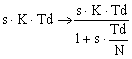﻿ 20-sim webhelp > Library > Signal > Control > PID Control > Derivative Control

# Derivative Control

With derivative action, the controller output is proportional to the rate of change of the measurement or error. Some manufacturers use the term rate or pre-act instead of derivative. Derivative, rate and pre-act are the same thing. The controller output is calculated by the rate of change of the error with time.

controller output = Td*d(error)/dt = Td*d(SP - PV)/dt

where the parameter Td is called derivative time. Derivative control is mathematically the opposite of integral action, but while we might have an integral-only controller, we would never have a derivative-only controller. The reason for this is that derivative control only knows the error is changing. It does not know what the setpoint actually is.

Derivative action has the potential to improve performance when sudden changes in measured variable occur, but is should be used with care. It is mostly a matter of using enough, not too much.

## Derivative Gain Limitation

In most commercial processes sudden changes in process output may appear. In most cases a sudden change in the slope of such a process output cannot be avoided at all times. Using such a process output in controllers with pure derivative action, would lead to unwanted steps in the controller output. Moreover, high frequency noise in the measured signals may lead to unwanted large outputs of the controller.

To prevent this unwanted effect, the derivative action can be filtered by a first-order system with time constant Td/N.This approximation acts as a derivative for low-frequency signal components. The gain, however, is limited to K*N. This means that high-frequency measurement noise is amplified at most by a factor KN. This is why the parameter N is called the derivative gain limitation. Typical values of N are 8 to 20. Sometimes the reciprocal value of N is used, mostly with the name beta (beta = 1/N).